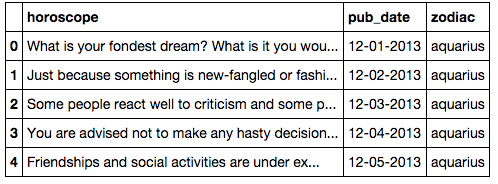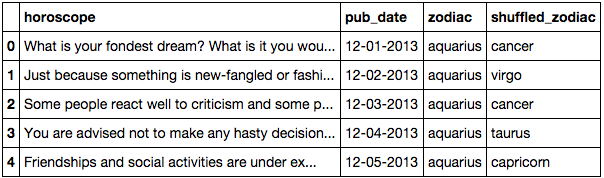# Modeling Horoscope Language

In the last post, I described my efforts at grabbing some data from a web site. Specifically, I scraped daily horoscopes from NYpost for a period of about a year and a half. While I have an admittedly poor understanding of astrology, I do know that each astrological sign is supposed confer some unique dispositional qualities to anyone born under that sign. If that’s true, then we would expect that the astrological readings would reflect the uniqueness of each sign. To be more explicit, the language found in a horoscope for each sign should carry some information about which sign it is most likely to belong to.

This is something that we can evaluate using the data I collected previously. In order to do so, we’re going to use a couple of supervised learning methods.

Friedman, Tibshirani & Hastie, in their widely used text The Elements of Statistical Learning describe machine learning algorithms with an analogy that I think works pretty well. The compare a supervised learning algorithm to a student in a classroom. For the student to learn something, the teacher presents him or her with some example problems along with the answers. The student learns the relationship between the problems and answers, and then applies what it has learned to some new problems, attempting to guess the correct answer.

In this analogy, the problems are the data and the answers are the labels or categories that each observation belongs to. For our dataset, the data are the individual horoscopes readings and the labels are the horoscope types that each corresponds to.

First, we’ll read in the data and look at the first few rows.The python module scikit-learn makes implementing a classifier fairly straightforward. First, we have to quantify all this text. That is, we have to create a numeric model of our language (i.e. a language model). One of the most straightforward ways of doing this is by creating a document-term matrix. This matrix features one row for each individual document and one column for each word that appears in the accumulated corpus of all the documents.

The values of the cells of this matrix can take on a number of forms. We’ll use a simple count of the number of times a given word appears in a given document.

In scikit learn, all this is done in two short lines.

So that’s four lines, but one is just importing the module and the last line is just to display what we’ve done.

We can see that this matrix has a total of 224,419 non-zero elements. That may seem like a lot until you consider that our matrix has 6271 rows (one for each horoscope) and 5505 columns (one for each word type), making for a total of about 34.5 million cells. This tells us that there are a whole lot of zeros in this matrix. This is typical for a language model like this.

So this forms our basic language model. We can use this simplistic representation to see if we can train a learner to distinguish between horoscopes from different astrological signs. For our first classifier, let’s see how a support vector machine does. We’re going to train the classifier on 70% of our data, holding out the remaining 30% to test it’s knowledge of the relationship between the text and the zodiac labels in the same way you would wait to show a student some examples until the final exam.

Now that we’ve fit a model, we can give this model some new data and tell it to give us its predictions. In other words, we’re going to give the model some new horoscopes and see how well it can place them as Aquarius or Pisces or what have you.

Okay, so this is relatively straightforward. We see the 12 signs down the left- hand side. The next column is the precision for each sign. Precision is a score that tells us how well the classifier identifies a category when it tries to do so. So, we see a score of 9% for aquarius. This means that, if the classifier labeled 100 horoscopes as ‘aquarius’, it was correct on 9 of those guesses.

Recall tells us how well the classifier does at identifying horoscopes from a given astrological sign. So, if there are 100 horoscopes labeled as ‘aries’, the classifier correctly guessed 14 of those.

The f1 score is the average of precision and recall, while support tells us how many instances of that category were in the test set. So, the average of precision and recall for capricorn is .08, while the last column tells us that there were 148 instances of capricorn in our test set.

What does this mean? Well, it doesn’t seem like we’re doing very well, does it? To give us some kind of comparison, what happens if we shuffle the zodiac labells such that they’re randomly assigned to different horoscopes? In principle, there should then be no relationship between a horoscope and the shuffled label. In other words, this would give us a way to evaluate what chance performance looks like.Okay, so I guess we can conclude that there’s no evidence that horoscope readings contain any information unique to the astrological sign that they come from, as this classifier basically performs identically to the one without shuffling the labels.

However, maybe this is a problem with the classifier we’ve chosen? It seems unlikely, but the cost to try another one is pretty low, so let’s give it a shot. We’ll use an ensemble method known as a random forest classifier to see if we can improve above chance. The implementation is not so different from the SVM we just tried.

I wont bother with shuffling the data, as we already know what chance performance looks like - the type of classifier wont change that.

So I think it’s safe to say that in this dataset, the language is uninformative of zodiac sign. However, maybe the language can tell us something else! Stay tuned - next time I’ll show that even if there’s no information in the language of astrological forecasts that tell us about the sign they came from, that doesn’t mean that they’re completely devoid of information. We’ll try to predict something else!

Written on July 16, 2015